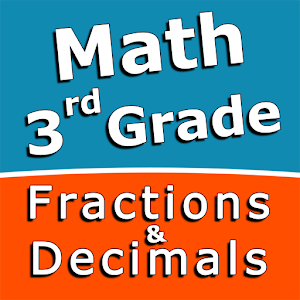Español

Todos los días ofrecemos apliaciones y juegos licenciados GRATIS para Android de otra manera tendrás que comprarlo.\$2.99
EXPIRÓ

# Android Giveaway of the Day - Third grade Math skills - Fractions and Decimals

Practice and improve the math skills!
\$2.99 EXPIRÓ
Votación de Usuario: 1 (100%) 0 (0%)

Esta oferta de regalo ha expirado. Third grade Math skills - Fractions and Decimals ya está disponible.

Intuitive interface powered by handwriting input and a choice of three fun and engaging mini games in addition to a regular math trainer mode make our app stand out from the crowd of generic math learning apps.

With Third grade Math skills - Fractions and Decimals you can practice and improve the following math skills:
- Add fractions with like denominators
- Subtract fractions with like denominators
- Write fractions in lowest terms
- Add decimal numbers
- Subtract decimal numbers
- Add three decimal numbers
- Convert decimals to fractions and mixed numbers
- Convert fractions and mixed numbers to decimals

Choose between four different modes:
- Math Trainer
- Math Shot mini-game
- Math Gravity mini-game
- Math Trick Shot mini-game

Education

### Tamaño: